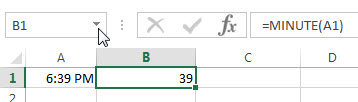# Excel MINUTE Function

This post will guide you how to use Excel MINUTE function with syntax and examples in Microsoft excel.

Description

The Excel MINUTE function returns the minutes of a time value. And the minutes is an integer number from 0 to 59. So you can use the MINUTE function to extract the minute component of a given time value.

For example, for a valid time value 10:29 AM, the MINUTE function will return 29. So you can pass the returned result into another formula.

The MINUTE function is a build-in function in Microsoft Excel and it is categorized as a DATE and TIME Function.

The MINUTE function is available in Excel 2016, Excel 2013, Excel 2010, Excel 2007, Excel 2003, Excel XP, Excel 2000, Excel 2011 for Mac.

### Syntax

The syntax of the MINUTE function is as below:

`=MINUTE (serial_number)`

Where the MINUTE function arguments is:

Serial_number -This is a required argument. A valid time value that contains the minute you want to extract. The times can be entered as a text strings within quotation marks (for example, “10:32 AM“), or typed as a decimal numbers, or the results of others formulas.

Note:

• Time values are a portion of a date value and represented by a decimal number (for example, 12:00 PM is represented as 0.5, since it is half of a day).
• If the serial_number is not a valid Excel time, it will return #VALUE! Error.

### Excel MINUTE Function Examples

The below examples will show you how to use Excel MINUTE Function to return an integer number that representing the minute component of a given Excel time.

1# get minute portion of the time, enter the following formula in Cell B1.

`=MINUTE(A1)`### Related Functions

• Excel HOUR Function
The Excel HOUR function returns the hour of a time value. Or returns an integer value that represent the hour component of a given time. And the return value is between 0-23.The syntax of the HOUR function is as below:=HOUR (serial_number)…
• Excel SECOND Function
The Excel SECOND function returns the seconds of a time value. Or returns an integer value that represent the second component of a given excel time. And the return value is between 0-59.The syntax of the SECOND function is as below:=SECOND (serial_number)…
• Excel DAY function
The Excel DAY function returns a day of a date (from 1 to 31). The DAY function is a build-in function in Microsoft Excel and it is categorized as a DATE and TIME Function.The syntax of the DAY function is as below:= DAY (date_value)
• Excel YEAR function
The Excel YEAR function returns a four-digit year from a given date value, the year is returned as an integer ranging from 1900 to 9999. The syntax of the YEAR function is as below:=YEAR (serial_number)…
• Excel MONTH function
The Excel MONTH function returns the month of a date represented by a serial number. And the month is an integer number from 1 to 12. The syntax of the MONTH function is as below:=MONTH (serial_number)…
• Excel TIMEVALUE Function
The Excel TIMEVALUE function returns the decimal number of the time represented by a text string. so it will convert a time represented by a text string into an Excel time value.The syntax of the TIMEVALUE function is as below:=TIMEVALUE (time_text)…